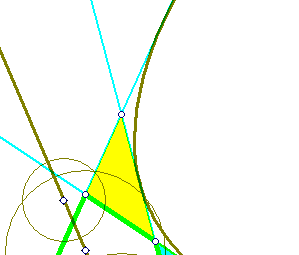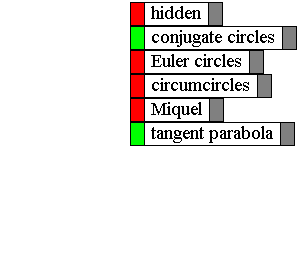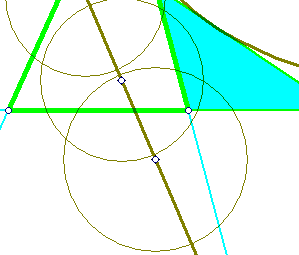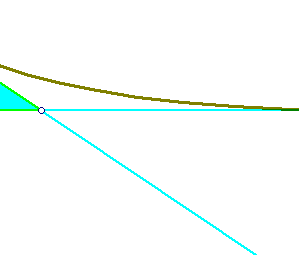##Impossible case to inscribe a rectangula hyperbola

Problem: Construct all rectangular hyperbolas tangent to four given lines determined by the sides of a quadrilateral q = A1A2A3A4 (may be convex or not).
The problem admits either two solutions or no solution at all. Since a triangle whose sides are tangent to a rectangular hyperbola must be obtuse-angled, the problem has solutions only if all partial triangles formed out of the four given points are obtuse-angled.
By partial triangles I mean the four triangles formed by selecting three sides of q. The picture below shows an impossible case. Two out of the four partial triangles are not obtuse-angled.The picture shows also the conjugate circles of the four partial triangles. Their centers are at the orthocenters of the partial triangles. The parabola tangent to the sides of q is also shown. See the file RectHyperbolasTangent4Lines.html for the discussion of the cases where the construction of the hyperbolas is possible.

Euler.html
EulerCircleProperty.html
FourEulerCircles.html
HyperbolaRectangular.html
NinePointsConic.html
OrthoRectangular.html
RectHypeCircumscribed.html
RectHyperbola.html
RectHyperbolasTangent4Lines.html
RectHypeThroughFourPts.html